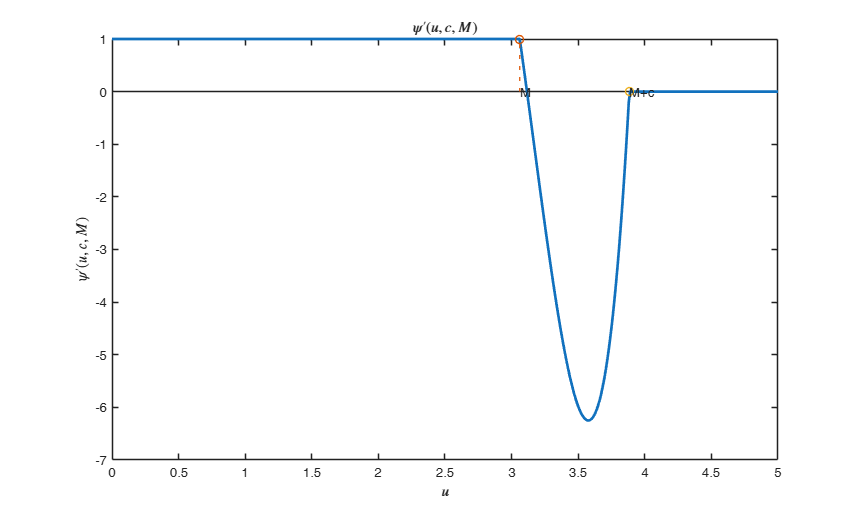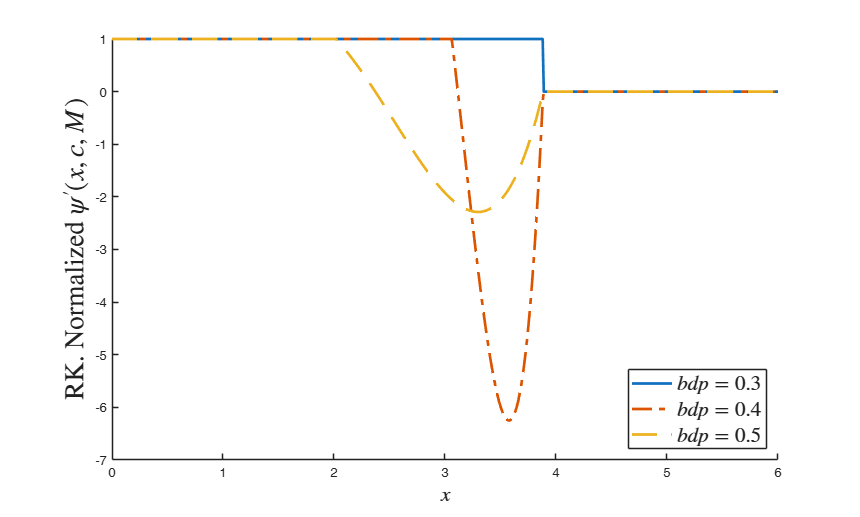# RKpsider

RKpsider computes derivative of psi function (second derivative of rho function) for Rocke (translated Tukey's) biweight

## Syntax

• psiderRK=RKpsider(u,c, M)example

## Description

 psiderRK =RKpsider(u, c, M) Plot of psider function.

## Examples

expand all

###Plot of psider function.

close all
% Find the values of c and M given bdp=0.4 and v=5 for ARP=0.01
x=0:0.01:5;
bdp=0.4;
v=5;
ARP=0.01;
[c,M]=RKbdp(bdp,v,ARP);
psiderRK=RKpsider(x,c,M);
% psiderRK=psiderRK/max(psiderRK);
plot(x,psiderRK,'LineWidth',2)
xlabel('$u$','Interpreter','Latex')
ylabel('$\psi'' (u,c,M)$','Interpreter','Latex')
title('$\psi'' (u,c,M)$','Interpreter','Latex')
hold('on')
stem(M,1,'LineStyle',':','LineWidth',1)
text(M,0,'M')
stem(M+c,0,'LineStyle',':','LineWidth',1)
text(M+c,0,'M+c')## Related Examples

expand all

###Compare Rocke psider function for 3 different values of bdp.

close all
x=0:0.01:6;
% Number of variables v is fixed to 5
v=5;
% ARP is fixed to 0.01
ARP=0.01;
lwd=2;
hold('on')
% Use bdp=0.3
bdp=0.3;
[c,M]=RKbdp(bdp,v,ARP);
psiderRK030=RKpsider(x,c,M);
plot(x,psiderRK030,'LineStyle','-','LineWidth',lwd)
% Use bdp=0.4
bdp=0.4;
[c,M]=RKbdp(bdp,v,ARP);
psiderRK040=RKpsider(x,c,M);
plot(x,psiderRK040,'LineStyle','-.','LineWidth',lwd)
% Use bdp=0.5
bdp=0.5;
[c,M]=RKbdp(bdp,v,ARP);
psiderRK050=RKpsider(x,c,M);
plot(x,psiderRK050,'LineStyle','--','LineWidth',lwd)
xlabel('$x$','Interpreter','Latex','FontSize',16)
ylabel('RK. Normalized $\psi''(x,c,M)$','Interpreter','Latex','FontSize',20)
legend({'$bdp=0.3$', '$bdp=0.4$' '$bdp=0.5$'},'Interpreter','Latex','Location','SouthEast','FontSize',16)## Input Arguments

### u — scaled residuals or Mahalanobis distances. Vector.

n x 1 vector containing residuals or Mahalanobis distances for the n units of the sample

Data Types: single| double

### c — tuning parameter. Scalar.

Non negative scalar which (together with the other optional parameter M) controls the robustness/efficiency of the estimator (beta in regression or mu in the location case ...).

Data Types: single| double

### M — tuning parameter. Scalar.

Scalar greater than 0 which (together with the other optional parameter c) controls the robustness/efficiency of the estimator (beta in regression or mu in the location case ...).

Data Types: single| double

## Output Arguments

### psiderRK —Rocke psi (translated Tukey's biweight) associated to the residuals or Mahalanobis distances for the n units of the sample. n -by- 1 vector

$RKpsider(u)= \left\{ \begin{array}{cc} 1 & 1 \leq u \leq M \\ \left(1-\left( \frac{u-M}{c} \right)^2 \right)\left(1-\left( \frac{u-M}{c} \right)^2 -4u \frac{u-M}{c^2} \right) & M \leq u \leq M+c \\ 0 & u > M+c \\ \end{array} \right.$